﻿ QED g-factor is NOT successful theory !

# Feynman QED failed

## Quantum electrodynamics (= QED ) is "scam".

### [ QED combines quantum mechanics and Einstein relativity ? ]

(Fig.1)  ↓ Final quantum gravity is fantasy 10-dimensional string theory !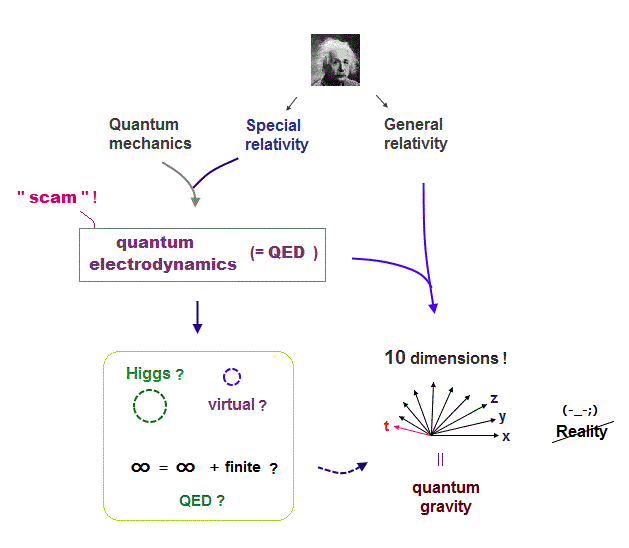They say that quantum electrodynamics (= QED ) is the most successful theory passing stringent tests.  But this boring cliche is a big lie.

QED is called relativistic quantum field theory combining quantum mechanics and Einstein relativity, though it relies on unreal virtual photons.

The only unified quantum gravity accepted now is string ( or M ) theory using unrealistic 10 spacetime dimensions.

So the present physics has reached a dead-end, meaning wrong and useless.

## QED is hocus-pocus, meaningless theory.

### [ QED uses artificial tricks to get convenient values. ]

(Fig.2)  Feynman and Dirac disbelieved QED !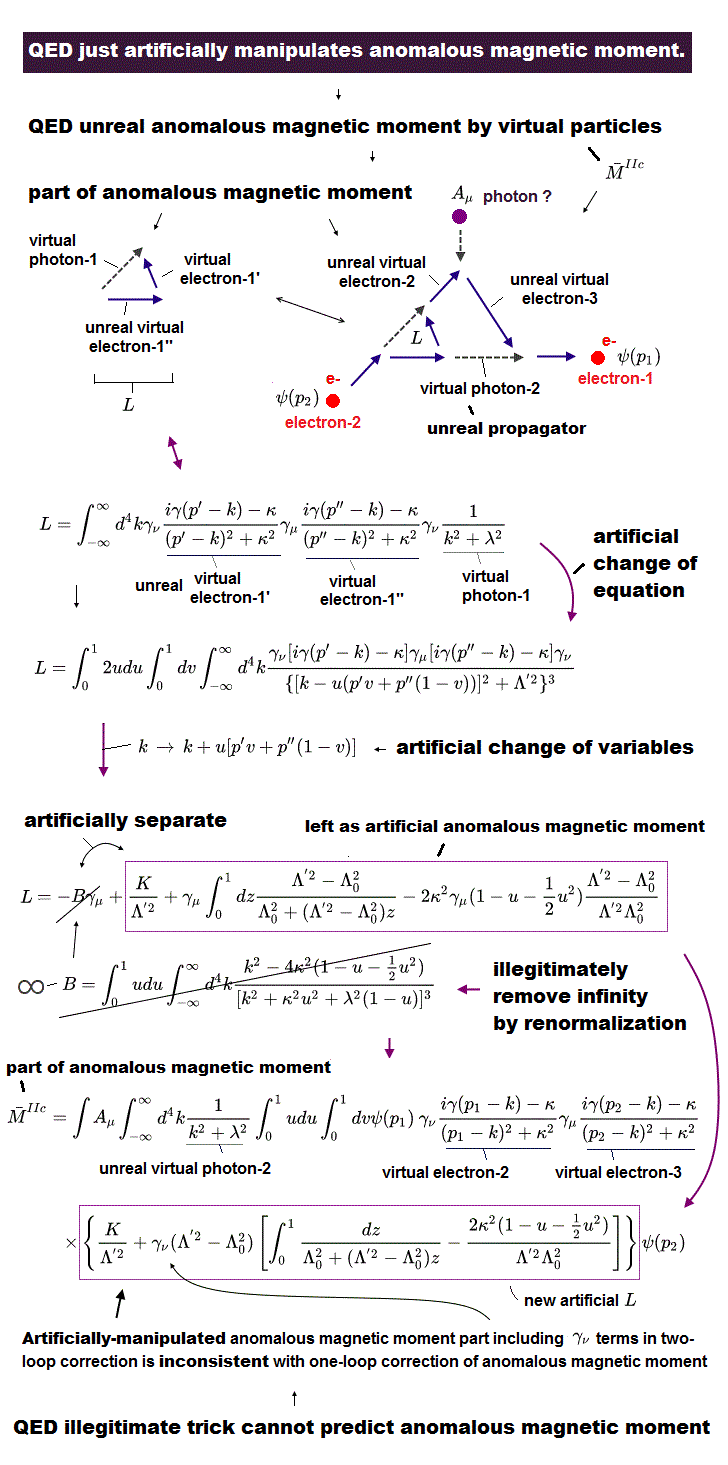Though universities and the media repeatedly say relativistic quantum theory such as QED and standard model are "triumph of the present physics !", it is a big lie.

Even its founders, Feynman and Dirac said "QED is hocus-pocus, mathematically NOT legitimate".

They are right ( so the present academic organizations are lying ).

## Remove "infinity" to get finite value ?

### [ QED renormalization eliminates infinity improperly ! ]

(Fig.3)  ↓ Infinity ∞ = infinity + "finite" value ? ← Nonsense"QED (= quantum electrodynamics ) is the most successful theory to predict unbelievably accurate physical values." is a big lie.

All calculations of QED always diverge to meaningless infinity by infinite number of unreal virtual particles with infinite energy or momentum surrounding electrons ( this p.3 ).

To remove the unrealistic infinite calculation results, QED started to say unrealistic things; "unmeasurable bare charge and mass of an electron are originally infinite ( this p.4 ), and these infinite bare charge and mass can artificially cancel out another infinite values caused by virtual particles (= self-evergy ) to get observed finite values ( this last,  this p.2 upper )."

This artificial trick of cancellation of infinity from another infinity to get desirable finite values (= anomalous magnetic moment or Lamb shift .. ) is called "renormalization", which illegitimate trick like "sweeping infinity under rug" is criticized even by QED founders.

↑ This means QED just artificially chooses some infinite values as fictitious (directly-unmeasurable) bare charge and mass of an electron and subtracts this chosen infinity from the QED calculated infinite values to get desirable finite values ( ∞ - ∞ = finite values, this p.2 ).

But in normal math, infinity minus infinity should be zero ( ∞ = ∞ = 0, because there is No upper limit in any infinite values ) or infinity (= when one of infinite values increases much faster than the other infinite value ).
Subtracting infinity from another infinity to get finite values is an illegitimate wrong math.

There is No legitimate rule or math validity in this unreasonable choice of arbitrary infinite values of bare charge and mass of an electron ( this, this ), and this fatal flaw is still unresolved even now.

For example, in calculating anomalous magnetic moment or electron's g-factor, QED uses illegitimate "change of variables (= changing infinite upper limit of virtual particle's energy into another infinite upper limit by an artificial finite value  → affecting the finite results after cancellation )."

This dirty trick is what QED relies on, hence, QED is just a wrong math, Not successful theory at all.

## QED depends on "absurd" tricks.

### [ QED method can change the original result intentionally. ]

(Fig.4)  Even when the original result is zero, QED can change it by force !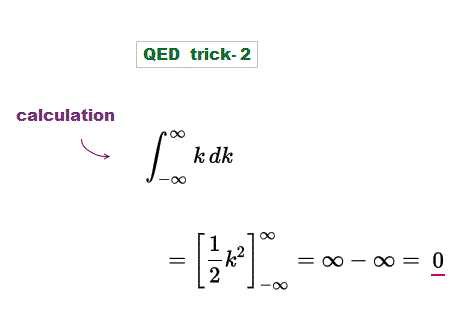Here we show one of QED unreasonable tricks.
In Fig.4, the original integral result is zero.

Because infinity minus infinity is zero ( ∞ - ∞ = 0 ).

## Change of variable = QED nonsense trick

### [ Change of variable can change the result in QED !? ]

(Fig.5)  Tricks using "change of variable"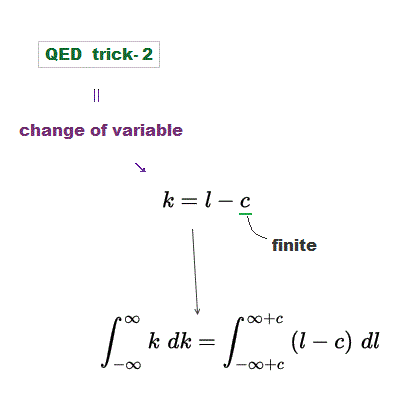One of the most important trick used in QED is "change of variable" in integrationa.  Change of variable is just a method, NOT changing the original result !

But "change of variable" of QED can change the calculation result artificially, which means QED relies on wrong math !

Here we replace "k" by "l-c" where l is variable, "c" is a finite value.

## Infinity = infinity ± finite value ?

### [ QED uses infinity as tool to get convenient value ! ]

(Fig.6)  ↓ Infinity (= ∞ ) absorbs any finite value (= c )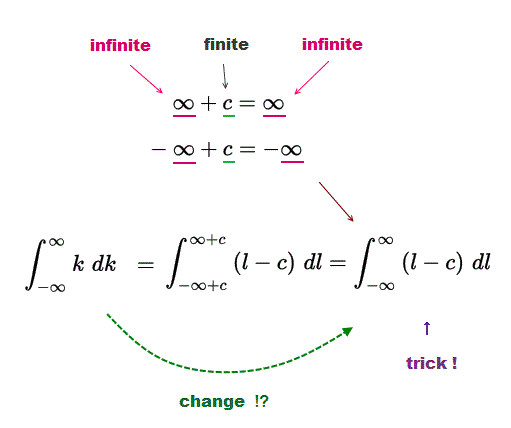This is a definite integral.  But its integral region is from -∞ to +∞.  Even if we translate this integral region by a finite value (= c ), it's meaningless.

Because ∞ + c = ∞ (= infinity remains infinity even after absorbing finite value c )  So the integral region is the same from -∞ to +∞ after change of variable.

## ∞ - ∞ = 0 ?

### [ But this infinity is NOT the same as "original infinity" ! ]

(Fig.7)   ↓ this calculation is correct ?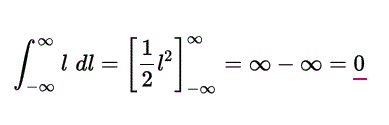Wee can apply the same integral used in the original result to this ?
In Fig.7 integration, ∞ - ∞ = 0, so the result is zero ?

## New finite value "c" remains !

### [ Change of variable in QED changes the original result ! ]

(Fig.8)  ↓ Artificial finite value c can be added to the original ?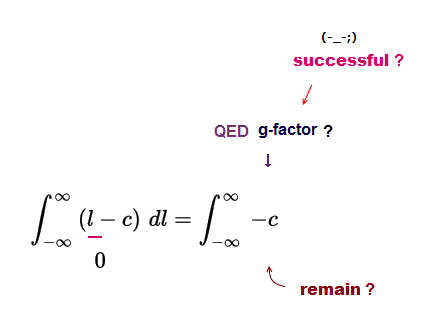As a result, this integral leaves the finite value "-c" used in this change of variable.  The original calculation result of this should be zero.

This means the absurd change of variable trick in QED can change the original result from "zero" to "-c" which is used as a spin g-factor !

This QED trick clearly violates the basic math rule, so wrong.

## QED absurd trick can change the original result !

### [ So QED is a meaningless theory which cannot predict any values ]

(Fig.9)  Zero → change of variable → -c !?    result changed ? = trick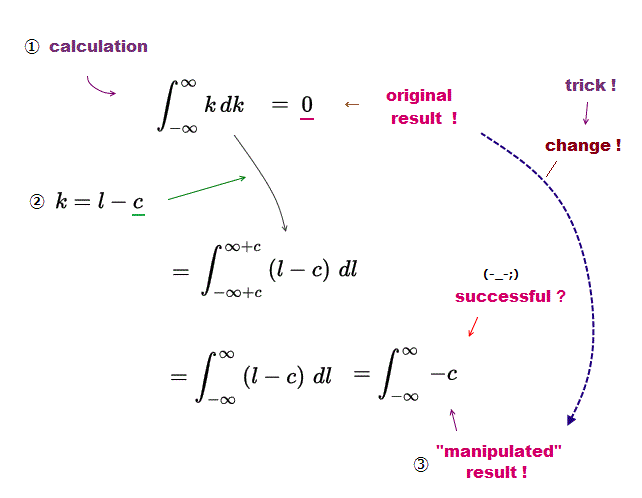The original result of this integral was zero (= ① )
But after change of variable (= ② ), the result changes from zero to "-c" (= ③ ) !

This is one of QED tricks to manipulate the calculation result !
SO QED depends on wrong artificial math calculation.

See other unreasonable tricks used in QED.

## QED is just "scam", NOT successful theory !

### [ Even founders disliked QED trick. ]

(Fig.10)  QED is hocus-pocus despite Nobel prize !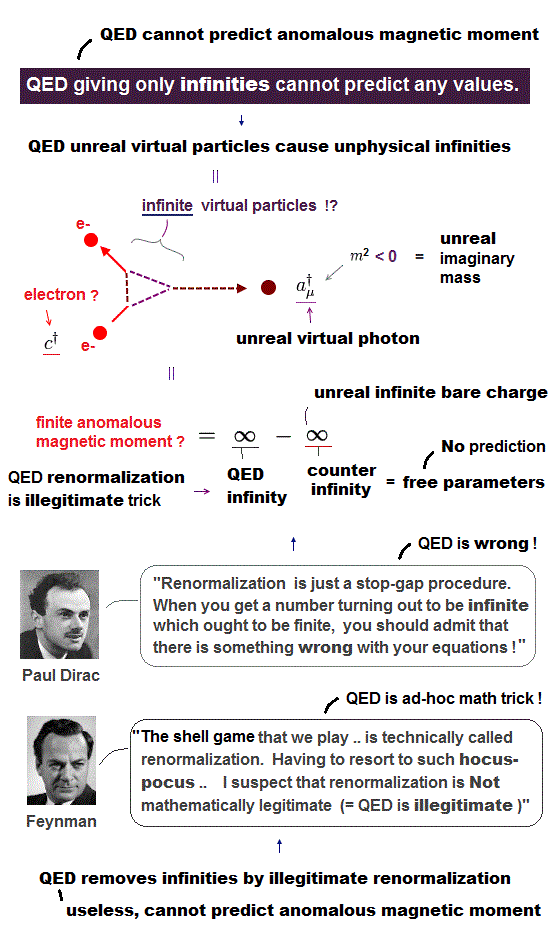Despite Nobel prize, QED is hocus-pocus, unreasonable math trick with NO scientific utility.

Actually this QED has made NO contribution to our society in any forms, except misleading and destroying young students' careers.

People and new students should notice they are deceived by universities which use Nobel prize as some "religion" to brainwash them into believing fictional science !2016/7/8 updated. Feel free to link to this site.